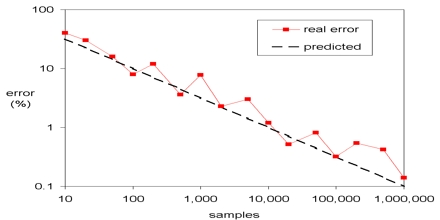Mathematic

# Monte Carlo MethodMonte Carlo method are a broad class of computational algorithms that rely on repeated random sampling to obtain numerical results. They are used in physical and mathematical problems and are most useful when it is difficult or impossible to use other mathematical methods. Monte Carlo method are mainly used in three distinct problem classes: optimization, numerical integration, and generating draws from a probability distribution.